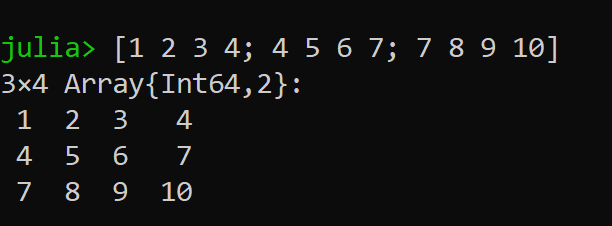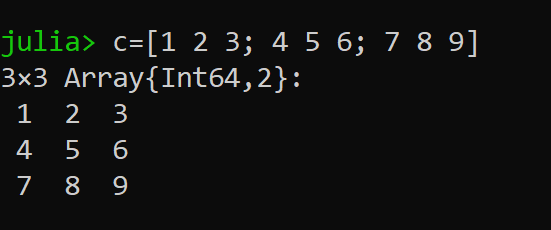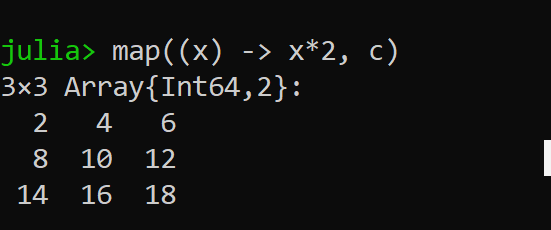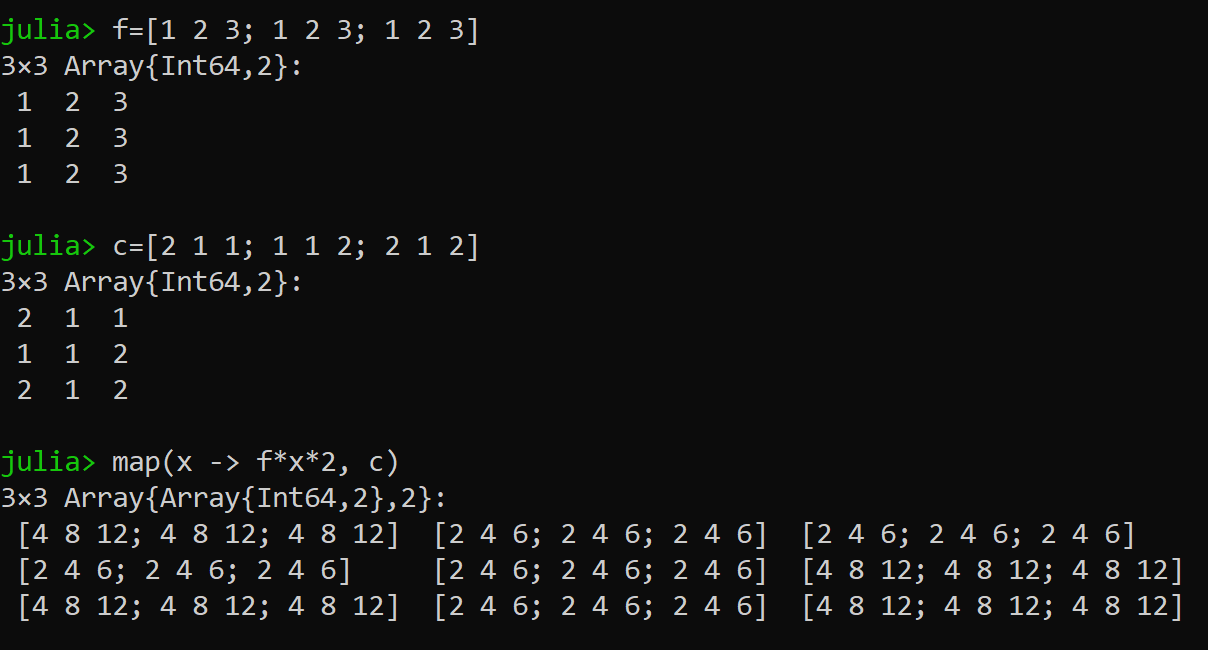Related Articles
Mapping over Matrices in Julia
• Difficulty Level : Expert
• Last Updated : 02 Jul, 2020

Julia is termed to be a fresh approach to Parallel Computing. Julia is a high-level and dynamic programming language for interactive use. Julia combines the implementation of scalar environments such as R and Python with the speed of dynamic programming languages like Java and C++ to provide a systematic solution for big data and analytics problems.

#### Julia Command Shell

As every programming language has its environment to develop dynamic models. Apparently Julia also features an interactive command shell REPL (REPL stands for read-eval-print-loop).

Example: Basic function
Input:

```Julia> a(x) = 2x^3+1; f(x, y) = 1 + a(x)y
Julia> println(“Hello World!”, “ I’m “, f(2, 1), “Welcome to Julia!”)
```

Here, We’ve declared a(x) where x is the parameter of the function f(x, y). We have allocated an operation  (2x^3+1) to a(x). Hence ” ^ ” operator performs multiplication. Now there is a function f(x, y) which gives us a result by performing a specific operation declared when parameter values are passed.

Output:

`Hello World! I’m 18 Welcome to Julia!`

#### Mapping over Matrices

Mapping the matrices in Julia is nothing but performing a function or an operation to each and every element in the matrix.

For example, Let us create a matrix in Julia and understand the terms in a lucid way.

```julia> variable = [1 2 3 4 5 6]
1×6 Array{Int64, 2}:
1  2  3  4  5  6
```

Here, we have created a 1×6 matrix(consists of 1 row and 6 columns). We can also create matrices of different dimensions and with varied range of datatypes(i.e float, int, double) without specifying the type while declaring the value.In the above picture, a matrix of order 3×4 of the integer data type is created.

##### Performing the map function:In the above picture, the code is executed in the Julia command line where I have created a matrix of order 3×3.We have performed the map function in the above picture to the matrix c. Here x is a variable which stores each and every value present in the matrix c.

• Let us consider the first element in the matrix ‘ c ‘ ( i.e, 1) which is now stored in x (x = 1).
• ( x -> x*2 ) explains us the variable x is assigning the value of x*2. Hence we explain it as (x = 1* 2)
• Exactly this operation is done to each individual value present in the matrix.
• Hence, we obtain the result after performing an operation using map function.
##### Performing the map function over two matrices:

In the above-explained examples, we have seen operations performed on a single matrix(i.e, c). Now let us understand the mapping technique by performing the operations on two different matrices.

```julia> f = [1 2 3; 1 2 3; 1 2 3]
3×3 Array{Int64, 2}:
1  2  3
1  2  3
1  2  3
```

Created a matrix ‘ f ‘of order 3×3.

```julia> c = [2 1 1; 1 1 2; 2 1 2]
3×3 Array{Int64, 2}:
2  1  1
1  1  2
2  1  2
```

Created a matrix ‘ c ‘of order 3×3.

```julia> map(x -> f*x*2, c)
3×3 Array{Array{Int64, 2}, 2}:
[4 8 12; 4 8 12; 4 8 12]  [2 4 6; 2 4 6; 2 4 6]  [2 4 6; 2 4 6; 2 4 6]
[2 4 6; 2 4 6; 2 4 6]     [2 4 6; 2 4 6; 2 4 6]  [4 8 12; 4 8 12; 4 8 12]
[4 8 12; 4 8 12; 4 8 12]  [2 4 6; 2 4 6; 2 4 6]  [4 8 12; 4 8 12; 4 8 12]
```
• We’ve used the map function to perform an operation on the matrices(f & c).
• x is a variable which stores every single value of c per operation.
• Now let’s try to understand the logic in the above code.
• Consider the first value in ‘ c ‘ matrix which is considered to be ‘ 2 ‘.
• The integer ‘ 2 ‘ which is the first element in the matrix c is now stored in x (i.e, (x -> f*x*2 ) == (x = f*2*2) )
• The very next operation is to multiply each and every element of matrix ‘ f ‘ to the above mentioned step (x = f*2*2)
• Here, we can see the first element in the matrix ‘ c ‘(i.e, 2) multiplied with all the values of matrix ‘ f ‘ in a row wise manner.
• Apparently, after multiplying the very first element in matrix ‘ c ‘(i.e, 2). Now the compiler shifts the value of ‘ x ‘ to the very next value (row wise consideration) present in matrix ‘ c ‘ (i.e, 1)
• Hence, (i.e, (x -> f*x*2 ) == (x = f*2*2) ) becomes (i.e, (x -> f*x*2 ) == (x = f*1*2) ). and every element in matrix ‘ f ‘ will be multiplied with value ‘ 1 ‘
• The same process repeats so on and so forth.The final executed output image.

Conclusion:

Hence, we now got to know what is a mapping function in Julia and what operations are performed and the executed using this function in Julia. We also executed the function to map over the matrices.

My Personal Notes arrow_drop_up
Recommended Articles
Page :